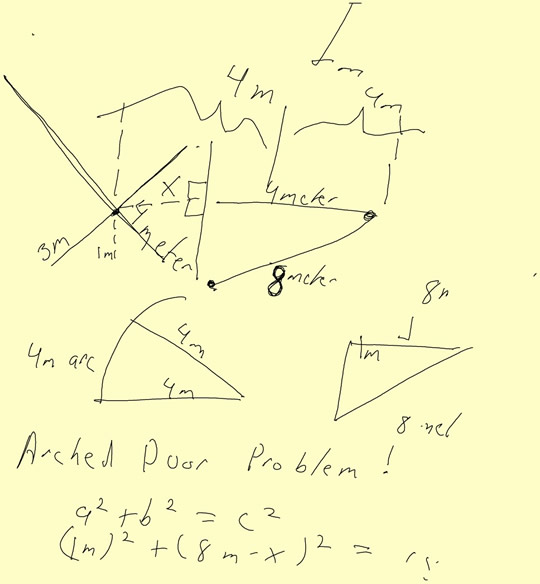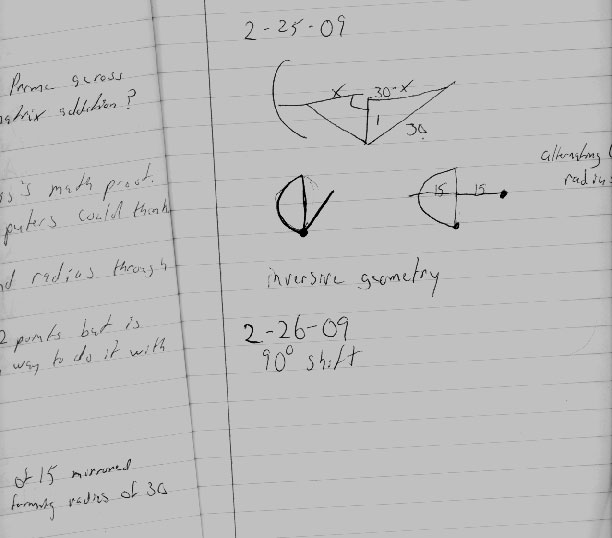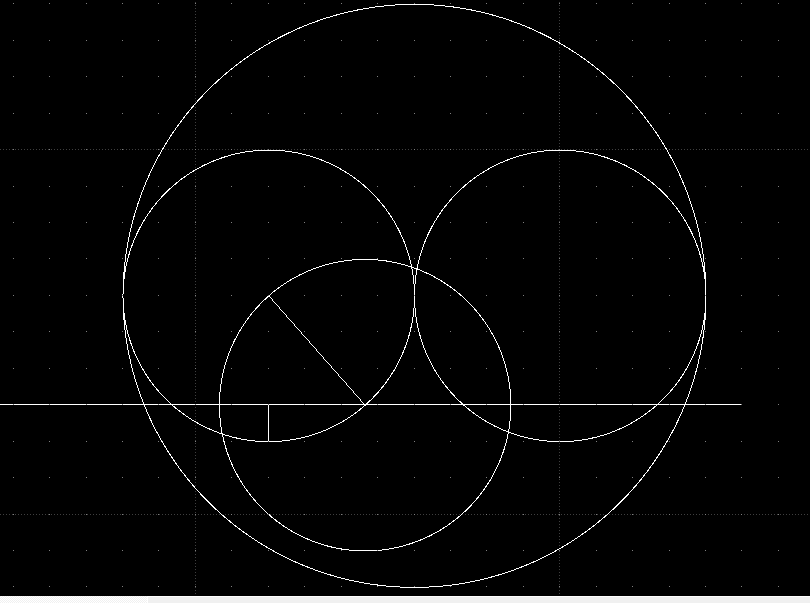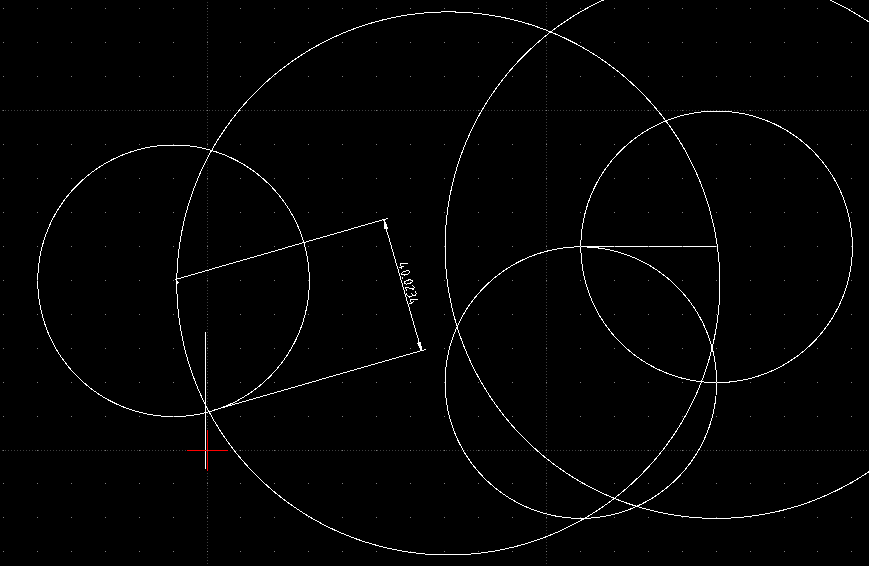_______ 20090211-0226 For this problem refer to Ladder vs Alley problem. The following is a preliminary sketch of the “Ladders in Alley” problem which was introduced for me to solve by my good friend C. Blanco. My solution is not presented in a formal mathematical way. Also my notes are quite messy. Hopefully I will be able to describe my approach. It is important to note that this work is incomplete and as such may have many errors. There is a type of geometry known as inversive geometry. I am not familiar with the subject. However the important basis to this work deals with the fact that the circular arc has an inverse. Think of a mirror placed between the arc that is formed by the 4 meter ladder. We use the base of the ladder as the radius through which the ladder rotates. There lies a radius which will intersects two points on the ladder. Here I doubled the radius from 4 to 8. But I don’t think it would matter what the mirrored length is as long as it is consistent between the two points. Then it is just a matter of finding the length of the 4 meter ladder corresponds to the 1 meter height. I do not solve the complete problem here, since once this triangle is determined the problem is easily solved using trigonometry. Review the Arched Doorway ProblemMay the Creative Force be with You